You have no items in your shopping cart.

\$USD
\$# VEX Robotics DC Motor Introduction

Motion is an integral part of mobile robotics. Whether robots are lifting an object, spinning a wheel, or performing some other action, they almost always include some form of actuator. The most common way to achieve such motion is through the use of a motor, and the most common type of motor used in hobbyist or competition robotics is the direct current (“DC”) motor.

DC motors, at their core, operate under a straightforward principle: the conversion of electrical energy (an input voltage and drawn current) into mechanical energy (an output shaft spinning at some speed, with some amount of torque).

The amount of mechanical output produced by a motor is dependent upon the amount of load placed upon it. This concept is key to understanding DC motor performance. As load (or mechanical resistance) increases, motors will draw more current and output more torque to overcome this load. However, as that torque increases, speed decreases proportionally. Additionally, as current draw increases, battery life decreases and wear on the motor increases.

To learn more about the relationships between speed, torque, and power, visit Unit F.4 of the VEX IQ Curriculum or Unit 7.2 of the VEX EDR Curriculum.

For a more in-depth look at DC motors and their use in robotics, visit Unit 7.3 of the VEX EDR Curriculum.

## The Four Key Characteristics of DC Motors (top)

These tradeoffs must all be considered when designing a mechanism that uses a motor in a particular application. To help understand a specific motor’s capabilities in such an application, four key characteristics are used to define a motor’s performance:

• Free Speed (RPM) – The maximum speed at which a motor will rotate when under no load
• Free Current (A) – The minimum current a motor will draw when under no load
• Stall Torque (N ∙ m) – The amount of load placed on a motor that will cause it to stall (stop moving)
• Stall Current (A) – The amount of current drawn by a motor when it is stalled

These characteristics represent the theoretical minimum and maximum conditions for a motor. Under no load, a motor spins at its Free Speed and draws a Free Current. Conversely, Stall Torque and Stall Current represent a motor being worked to its maximum, to the point at which it can no longer move.

In good mechanism design, motors rarely operate at one of these four edge conditions. Holding something at stall torque (with max input voltage) is a great way to quickly destroy a motor, and spinning at free speed ensures that very little actual work can be done (since max speed corresponds to zero torque). Instead, engineers use motor curves to optimize motor usage somewhere in the middle.

### The "Down-Up" Dyno Test (top)

VEX Robotics motor curves were developed experimentally using a “down-up” dynamometer test.

1. A motor is spun at free speed
2. A brake is slowly applied (linearly increasing in torque over time), bringing the motor down to a predetermined RPM
3. The brake is slowly released and the motor is allowed to return to its free speed

A variety of data, such as output speed, output torque, current draw, and power input/output, is taken throughout this test. The “down” (brake applied) side is then averaged with the “up” (brake released) side.

### Why "Down-Up"?

The mechanics of a dyno test are crucial to developing and publishing accurate motor specifications. When a motor is spinning at free speed while attached to a dyno drum, the system contains a high amount of rotational inertia. This inertia complements the motor’s own output, creating a false reading for peak output power that can be higher than the motor’s actual performance.

However, manufacturers do not always adjust for inertia cancellation when measuring their motor’s performance, and spec sheets rarely detail the circumstances under which their information was derived.

By testing and averaging both the “down” (inertia helping the motor) and “up” (inertia resisting the motor) sides, this method is the best way to represent a motor’s true capacity.

## Watt Is Power (top)

As mentioned above, there are two types of power when talking about DC motors:

• Electrical Power (W or Win) - This is the amount of power (supplied voltage and drawn current) supplied to the motor.
• Mechanical Power (W or Wout) - This is the amount of work (speed with torque) the motor is producing. This is the power used on a motor curve.

Mechanical Power can be calculated using speed and torque: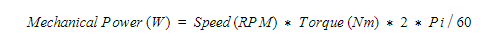A motor produces the most power at half of stall torque, or half of free speed. This point is called peak power. If you look at the motor curves on motors.vex.com, you want the motor to stay on the right side of peak power. Once you go past peak power the motor is drawing more electrical power to produce less mechanical power.

Power is arguably the most important characteristic of a motor since it characterized the amount of work a motor is capable of doing whereas speed and torque describe some of the work a motor is capable of doing.

When designing a mechanism you not only need it to do an action, but you want it done in a certain amount of time. Only using torque when selecting a motor will tell you that the motor is capable of doing that task, but not how fast the task can be done. Only using speed when selecting a motor will tell you the speed a task can be done, but not if the motor is capable of it.

Here are a couple of examples:

Example 1:

You have a lift driven by a 1.25” drum and the torque load is 20 Nm. You decide to use a motor that produces .2 Nm of torque at 3,000 RPM (62.8 W).

To produce enough torque, you’ll have to put a 100:1 reduction on the motor which reduces the speed down to 30RPM. On a 1.25” drum, the lift will move at approximately .16 in/sec.

Example 2:

You have the same lift in Example 1. This time you decide to use a motor that produces .2 Nm of torque at 10,000 RPM (209.4 W).

To produce enough torque, you’ll still need to use a 100:1 reduction on the motor. This reduces the speed down to 100 RPM. On a 1.25” drum, the lift will move at approximately .54 in/sec.

As you can see, even though both motors produce the same amount of torque, the motor used in Example 2 has over 3x the power because it is faster. Therefore, the lift will move over 3x faster than the lift in Example 1.

Since every robot action can be described as load (torque) moved in an amount of time (speed), you can easily figure out how much power is required to achieve that action. The amount of power is also unaffected by any reduction. This is because a reduction reduced speed and increased torque proportionally. This means that Power is also useful for not only helping you pick a motor, but telling you how many you need.

Here are some more examples:

Example 3:

You need to lift 25 lbs, 9 feet in the air in 1 second. Your team decides on a lift that is driven by a 4 inch diameter sprocket. Here’s how much power is required to achieve this:

To produce enough torque, you’ll still need to use a 100:1 reduction on the motor. This reduces the speed down to 100 RPM. On a 1.25” drum, the lift will move at approximately .54 in/sec.

1. Calculate how much torque is required in Nm.

The radius of the sprocket is 2": 4" / 2
A 25 lbs load on a 2in radius is 50 in-lbs of torque: 10 lbs * 2".
50 in-lbs is equal to 5.6 Nm: 50 in-lbs * .112 in-lbs/Nm

2. Calculate the speed required in RPM.

9 ft traveled over 1 seconds is p ft/s: 9ft / 1s
Converted to inches this would be 108 in/s: 9 ft * 12 in/ft
The circumference of a 4 inch diameter sprocket would be 12.56": 4" * Pi
The number of revolutions per second require is 8.59: 108" / 12.56in/revolution
8.59 rps is 515.4 RPM: 8.59 rps * 60 sec/minute

3. Calculate Power using the formula above

5.6 Nm * 515.4 RPM * 2 * Pi =302W

In this example, we’ll look at 2 different motors:
Motor Speed (RPM) Torque (Nm) Power (W)
A 15000 .1 157
B 5000 .2 104.7

5. Calculate How Many Motors Are Needed.

The application in this example requires about 302W of mechanical power. The motors listed above can produce 157W and 104.7W respectively.

(2x) of Motor A would need to be used: 302W required / 157W per Motor A = 1.92 Motors

(3x) of Motor B would need to be used: 302 W required / 104/7W per Motor B = 2.88 Motors

Now that you know the quantities of each motor, you can weigh the pros and cons of each motor to determine which to use.

Motors will never convert all the supplier electrical power to mechanical power. The rate the motor is converting electrical power to mechanical power is called Efficiency. Any electrical power that isn’t converted to mechanical power is dissipated at heat. The more power lost to heat, the hotter a motor will run.

Heat build up in a motor is bad since it affects the electrical resistance of the motor’s windings. As this changes, the efficiency of the motor gets even worse and will produce even more heat. This cycle continues until the motor is turned off and given a chance to cool down, or the motor permanently fails.

The point when a motor is most efficient is called peak efficiency. This is the point where the motor is converting the most amount of electrical power into mechanical power. Typically, this is the best point for a DC motor to operate.

## Using a Motor Curve (top)

Motor curves are primarily used in two scenarios: Determining what motor (and gear reduction) to use in a particular application, and learning more about the state of a motor currently operating in a system.

### 1 - Determining which motor (and gear reduction) to use in a particular application

#### "Which motor should I use to lift this object?"

In competition robotics, the task to be performed will need to be defined and modeled by the designer. As a simplified example, consider a game piece that weighs 40.0 N, being lifted by an arm that is 0.5 m long, running through a 100:1 gearbox: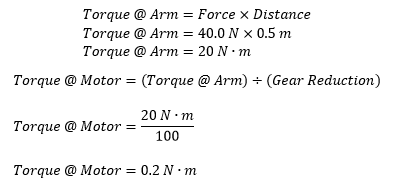Thus, the motor that drives this arm will need to output 0.2 N · m of torque.
Note: To learn more about how gear ratio can affect speed and torque, visit Unit G.3 of the VEX IQ Curriculum or Unit 8.4 of the VEX EDR Curriculum.

This output torque requirement can then be compared against published Motor Curves to learn more about the state of the motor during this action. Consider the following two curves [click to enlarge]: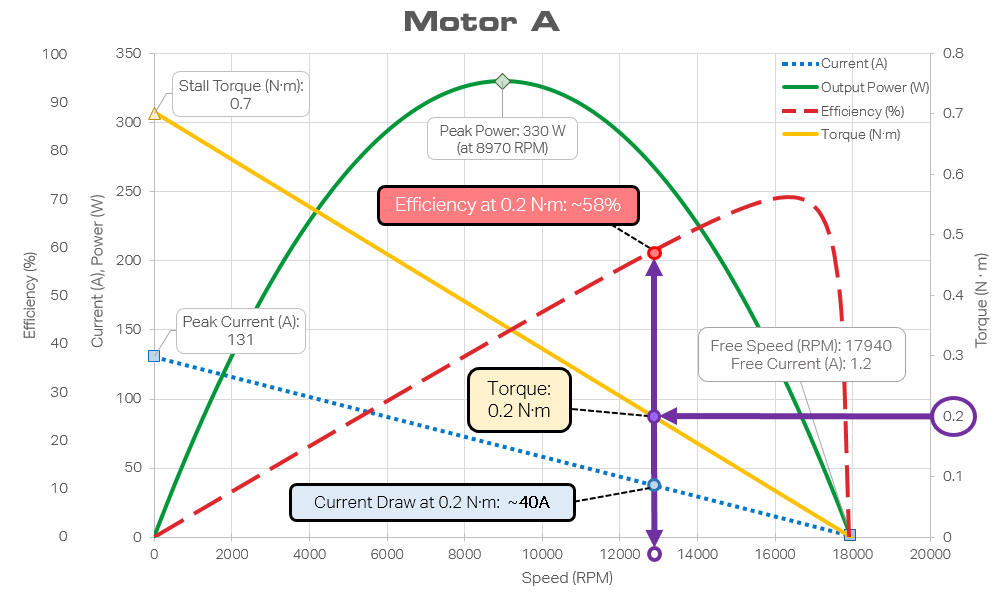Motor A achieves 0.2 N · m of torque at roughly 13000 RPM, drawing roughly 40 A.
With a 100:1 gearbox, this equates to an arm speed of 130 RPM.
At this torque, the motor is operating just under 60% efficiency, below its peak.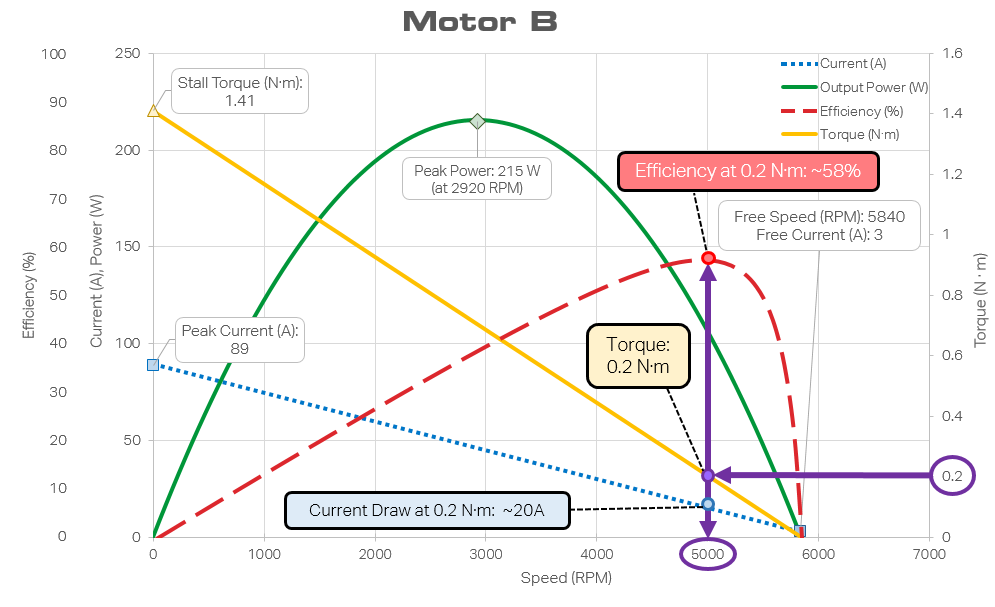Motor B can achieve 0.2 N · m of torque at roughly 5000 RPM, drawing roughly 20 A.
With a 100:1 gearbox, this equates to an arm speed of 50 RPM.
At this torque, the motor is operating at around 60% efficiency, very near its peak.

Using these types of calculations, an engineer can combine this information with other details of the system to determine the best motor for their application. Is 50 RPM too slow? Is 40 A too high of a current draw? Is a higher efficiency needed for thermal or battery reasons?

As should quickly become evident, there are a number of variables involved in making this determination. Gear ratio, arm length, even game piece weight. Many times, designers will develop spreadsheet calculators to modify these values and seek out a condition that satisfies their needs.

#### Note: Current Draw at Peak Power

For an even quicker "back of the napkin" calculation, designers will sometimes simply calculate the amount of work being performed (Work = Mass × Gravity × Height) over a set amount of time (Power = Work ÷ Time) and select a motor matching that power requirement. For example, if a robot had to lift a 20.0 kg object 1.0 m in the air over 1.0 second:In the above example, Motor B would be the closest match for a power requirement of 196 W.

However, what happens when there are two motors matching that peak power need? It is generally best to choose the motor with the lowest current draw, as this will extend your battery life and reduce strain on the electrical system. This becomes especially important under a sustained load, when current limiters or circuit breakers must be taken into account. This is covered in more detail below.

### 2 – Learning more about the state of a motor currently operating in a system

#### "Why does this motor burn up after lifting the object?”

If a motor has already been installed and an engineer wants to know more about the state of the system, the same theory as above can be reversed. At a known voltage, it takes one measured value (such as current draw) to determine the rest of the motor’s attributes at that moment.

For example, again consider Motor A. If an ammeter is used to measure a current draw of 25 A, then it is now known that the motor is exerting around 0.11 N · m of torque and is operating around its peak efficiency of just under 70%. However, if the ammeter is reading 140 A, then the motor is currently operating under an extreme stall condition.

Note: VEX Robotics motor curves were made at 12 V. The four key characteristics (free speed / current, stall torque / current) approximately scale proportionally with system voltage. If Motor A was running at 6 V, its stall current would drop from 130 A to 65 A, and its stall torque would drop from 0.7 N · m to 0.35 N · m. If an ammeter reading showed a current draw of 25 A: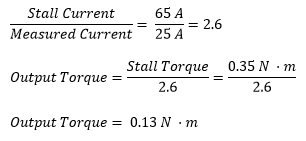## Other Considerations (top)

While motor curves are an immensely useful tool to use during mechanism design, there are a few other characteristics of a motor or a system to keep in mind.

### Thermal Mass

Most motors, if pushed to their stall torque or peak power, will burn themselves out if left there for too long. However, that acceptable time varies from motor to motor. Some motors may have a very high peak power, but can only be run under that full power in short bursts. Other motors have no problem sitting at their peak power, but may have other drawbacks (higher current draw, lower peak power, etc).

Because this property is so inherently dependent on the system and application, it is not typically published by manufacturers. However, thermal mass can be approximated by dividing a motor’s peak power by its weight. For example:

Peak Power Motor Weight Thermal Mass
Motor A 330 W 0.75 lb 440 W/lb
Motor B 215 W 2.16 lb 99.5 W/lb

In this scenario, Motor A would be more useful for shorter bursts of high power, while Motor B could sustain its power for much longer. However, this comparison should only be used as a relative approximation, not as a firmly defined property.

### Current Limits

Most competitive robots involve some sort of safety cutoff, such as a thermal cutoff inside the motor or a current limiting circuit breaker. These limits must be taken into account when determining an acceptable current draw for a given application.

Circuit breakers can usually hold a current draw past their rating for a set amount of time. Manufacturers typically provide a spec sheet that includes a graph such as the following, taken from the 20A / 30A Snap Action VB3 circuit breakers sold by VEXpro: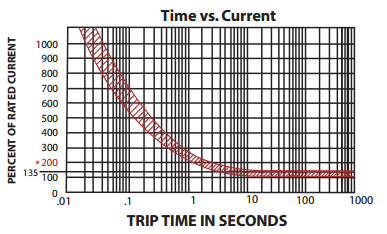If Motor A from the above example (drawing 40 A) were wired through a 20A breaker, it would be pulling 200% of the breaker’s rated current. Referencing this chart, the system could sustain this draw for roughly 1 – 4 seconds before tripping the breaker and cutting power to the motor.

### Mathematical Assumptions

Though the motor curves are experimentally built, the calculations they are being used for ignore many real-world system characteristics such as friction, shock loading, or the effects of gravity (such as when moving an arm up vs moving it down). In some cases, these assumptions lend themselves to a more conservative calculation; in some cases, the opposite is true.

It is important to remember that the engineering design process consists of much more than calculations based off of spec sheets and charts – once a direction has been set, it’s time to build a prototype, test, and iterate as required!